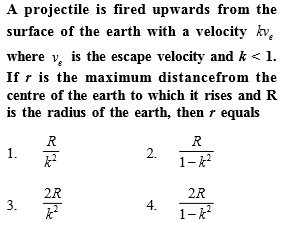# NEET Questions SolvedConcept Videos :-

#25 | Escape Velocity
#32 | Solved Problem Set 1
#33 | Solved Problems Set 2

Concept Questions :-

Escape velocity

$\left(2\right)\phantom{\rule{0ex}{0ex}}{K}_{i}+{U}_{i}={K}_{f}+{U}_{f}\phantom{\rule{0ex}{0ex}}\frac{1}{2}m{\left(k{v}_{e}\right)}^{2}-\frac{GMm}{R}=0-\frac{GMm}{r}\phantom{\rule{0ex}{0ex}}\frac{1}{2}m{\left(k\sqrt{\frac{2GM}{R}}\right)}^{2}-\frac{GMm}{R}=0-\frac{GMm}{r}\phantom{\rule{0ex}{0ex}}\frac{{k}^{2}}{R}-\frac{1}{R}=-\frac{1}{r}\phantom{\rule{0ex}{0ex}}r=\frac{R}{1-{k}^{2}}$

Difficulty Level:

• 15%
• 58%
• 18%
• 11%
Crack NEET with Online Course - Free Trial (Offer Valid Till September 23, 2019)